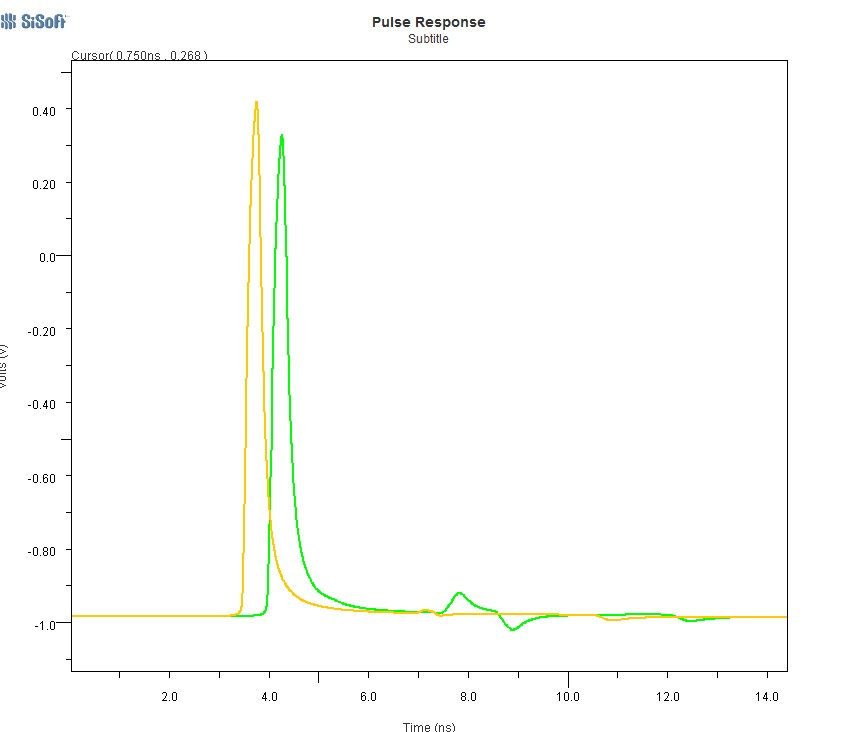# AR# 51825

## 描述

What are the requirements for the safe usage of Auto Adapting Mode in 7 series FPGAs GTX?

## 解决方案

A single bit response of the channel analysis in time domain is needed to decide if the adaptation algorithm can be used.

The adaptation algorithm performs better if the single bit response of the channel appears as a smooth exponential decay. If there are "deviations" from this smooth exponential decay (i.e., reflections due to impedance mismatches along the channel), a fixed CTLE is suggested.

In the image below, the comparison between a pulse response with smooth exponential decay (yellow curve) and one with a "deviation" due to impedance mismatch in the transmission line is shown (QCD calculates the impulse response in the Statistical analysis).deviation001.jpg
AR# 51825

Type 综合文章